# Geometry Basics Foundation

GCSEKS3Level 1-3AQACambridge iGCSEEdexcelEdexcel iGCSEOCRWJEC

## Geometry Basics: The 5 Simple Rules

Geometry basics will teach you the 5 simple rules needed to answer basic geometry questions, as well as give you the foundations to build as you work through the different geometry topics.

Having basic algebra knowledge is required to solve geometry problems.

Level 1-3GCSEKS3AQAEdexcelOCRWJECCambridge iGCSEEdexcel iGCSE

## Angles in a triangle add up to $180\degree$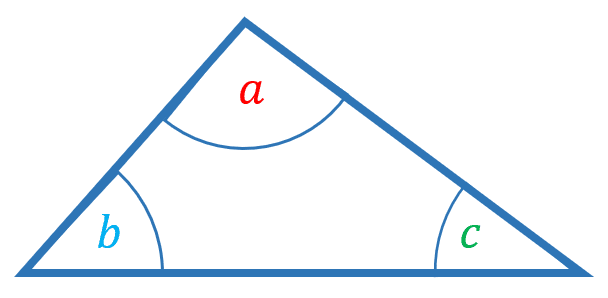The angles in a triangle add up to $180\degree$

$\textcolor{red}{a} + \textcolor{skyblue}{b} + \textcolor{green}{c} = 180\degree$

Level 1-3GCSEKS3AQAEdexcelOCRWJECCambridge iGCSEEdexcel iGCSE

## Angles in a quadrilateral add up to $360\degree$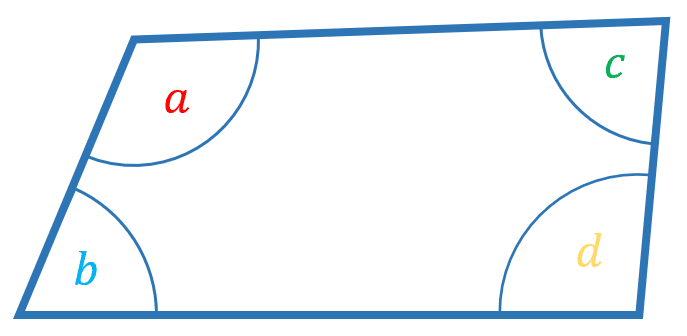The angles in a quadrilateral ($4$ sided shape) add up to $360\degree$

$\textcolor{red}{a} + \textcolor{skyblue}{b} + \textcolor{green}{c} + \textcolor{yellow}{d}= 360\degree$

Level 1-3GCSEKS3AQAEdexcelOCRWJECCambridge iGCSEEdexcel iGCSE

## Angles on a straight line add up to $180\degree$

The angles on a straight line all add up to $180\degree$

$\textcolor{red}{a} + \textcolor{skyblue}{b} + \textcolor{green}{c} = 180\degree$

Level 1-3GCSEKS3AQAEdexcelOCRWJECCambridge iGCSEEdexcel iGCSE

## Angles around a point add up to $360\degree$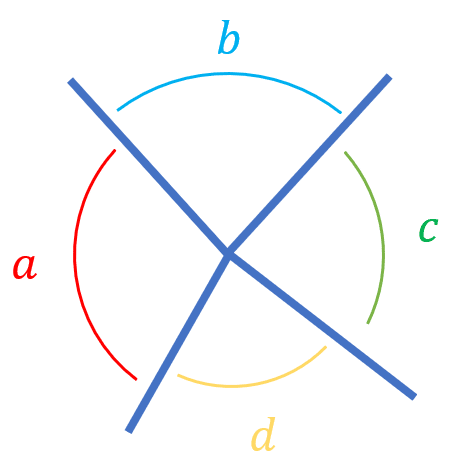The angles around a point all add up to $360\degree$

$\textcolor{red}{a} + \textcolor{skyblue}{b} + \textcolor{green}{c} + \textcolor{yellow}{d}= 360\degree$

Level 1-3GCSEKS3AQAEdexcelOCRWJEC

## Two sides and two angles of an isosceles triangle are the same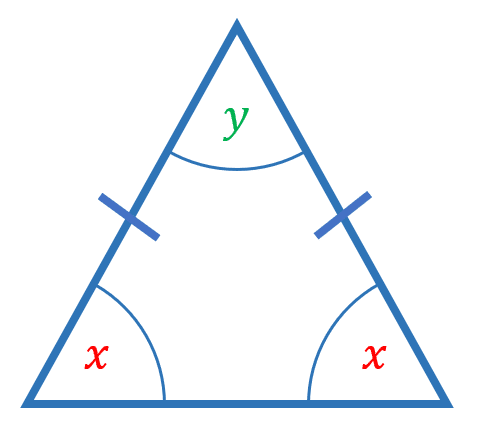The two sides marked with the lines are the same length.

The two base angles, $\textcolor{red}{x}$, are the same.

Level 1-3GCSEKS3AQAEdexcelOCRWJECCambridge iGCSEEdexcel iGCSE
Level 1-3GCSEKS3AQAEdexcelOCRWJECCambridge iGCSEEdexcel iGCSE

## Example 1: Angles in a Triangle

Find the value of $x$ in the triangle shown:

[2 marks]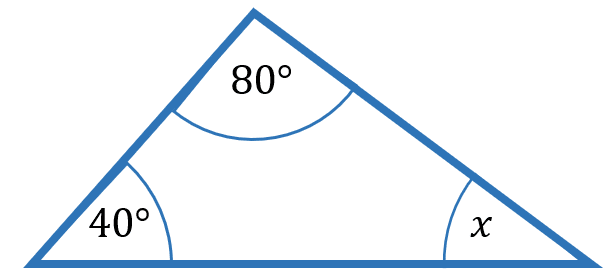We know that angles in a triangle add up to $180\degree$,

$40\degree + 80\degree + x\degree = 180\degree$

$x= 180\degree -40\degree - 80\degree = 60\degree$

$x= 60\degree$

Level 1-3GCSEKS3AQAEdexcelOCRWJECCambridge iGCSEEdexcel iGCSE

## Example 2: Finding a Missing Angle

Find the value of $x$ in the triangle shown:

[2 marks]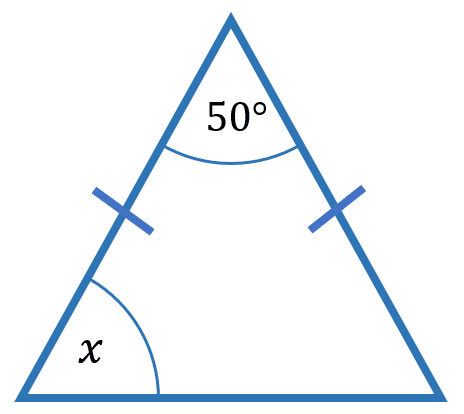We know that in an isosceles triangle, the base angles are equal.

This means we can form the equation:

$x\degree + x\degree + 50\degree = 180\degree$

$2x\degree = 180\degree - 50\degree$

$2x = 130\degree$

$x\degree = 65\degree$

Level 1-3GCSEKS3AQAEdexcelOCRWJECCambridge iGCSEEdexcel iGCSE

## Geometry Basics Foundation Example Questions

Angles on a straight line all add together to make $180\degree$

\begin{aligned}103\degree+\angle CDB &= 180\degree \\ \angle CDB &= 180\degree-103\degree = 77\degree \end{aligned}Gold Standard Education

Angles around a point all add together to make $360\degree$

$100\degree+50\degree+x\degree+105\degree =360\degree \\ x=360\degree-100\degree-105\degree-50\degree \\ x= 105\degree$Gold Standard Education

Base angles in an isosceles triangle are equal and angles in a triangle add up to $180\degree$

$61\degree+61\degree+y\degree =180\degree \\ y\degree =180\degree-61\degree-61\degree \\ y=58\degree$Gold Standard Education

Base angles in an isosceles triangle are equal and angles in a triangle add up to $180\degree$

\begin{aligned}x+x+55\degree&=180\degree \\ 2x&=180\degree-55\degree = 125\degree \\ x&=\dfrac{125\degree}{2}\end{aligned}

$x=62.5\degree$Gold Standard Education

Angles on a straight line all add together to make $180\degree$ so

$x=180\degree-115\degree=65\degree$

Angles in a triangle add up to $180\degree$

$y=180\degree-25\degree-65\degree$

$y=90\degree$Gold Standard Education

## Geometry Basics Foundation Worksheet and Example Questions

### (NEW) Geometry Problems Foundation Exam Style Questions - MME

Level 1-3GCSENewOfficial MME

## Geometry Basics Foundation Drill Questions

Level 1-3GCSE

### Geometry Problems - Drill Questions

Level 4-5GCSEProduct